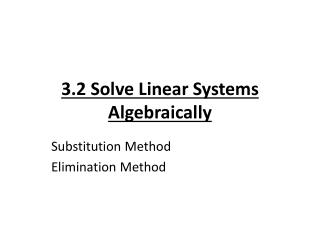DownloadDownload Presentation3.2 Solve Linear Systems Algebraically

# 3.2 Solve Linear Systems Algebraically

Download Presentation## 3.2 Solve Linear Systems Algebraically

- - - - - - - - - - - - - - - - - - - - - - - - - - - E N D - - - - - - - - - - - - - - - - - - - - - - - - - - -
##### Presentation Transcript

1. 3.2 Solve Linear Systems Algebraically Substitution Method Elimination Method

2. Substitution Method • 3 Steps: 1) Solve one of the equations for one of its variables 2) Substitute the expression from Step 1 into the other equation and solve for the other variable 3) Substitute the value form Step 1 into the revised equation from Step 1.

3. Solve the system using the substitution method Eq 1: 2x + 5y = -5 Eq 2: x + 3y = 3 Step 1: Solve the equation for x in Eq 2. x + 3y = 3 x = -3y + 3 Step 2: Substitute the expression (-3y + 3) into Eq 1 for the variable x. 2 ( ) + 5y = -5 Solve for y: Step 3: Substitute the y value into the revised Eq. (Eq 2)

4. Practice Problem: • Ex1) 4x + 3y = -2 x + 5y = -9

5. Elimination Method Type the Steps from Page 161. Step 1: Step 2: Step 3:

6. Use the elimination method: Ex 3) 3x – 7y = 10 6x – 8y = 8 Step 1: Multiply (Eq 1) by a -2 Step 2: Add the revised Equations and solve for y. Step 3: Substitute the value y in for one of the original equations. Solve for x.

7. Practice Problem: • Ex) 3x + 3y = -15 5x – 9y = 3